# Birkhoff normal form

(diff) ← Older revision | Latest revision (diff) | Newer revision → (diff)

Birkhoff–Gustavson normal form

Usually, a formal normal form (cf. Normal form of a system of differential equations) for a time-independent Hamiltonian system in the neighbourhood of a stationary point (cf. Normal form in a neighbourhood of a fixed point) for which the linearized system at the stationary point has only purely imaginary eigenvalues.

Consider a Hamiltonian system on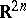with Hamiltonian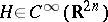, i.e.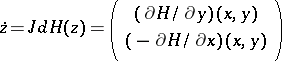with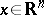,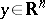,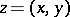,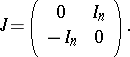Suppose that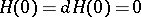. The origin is a stationary point and the Hamiltonian evaluated at the origin iswhere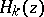denotes the homogeneous terms of degree. Furthermore, suppose that the matrix of the linearized system,, is diagonalizable (over) with purely imaginary eigenvalues,,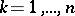. Let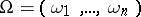. The eigenvalues are called resonant if they are rationally dependent, i.e. if there is an integer-valued vector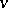such that, whereis the standard inner product on. The eigenvalues are non-resonant if there is no such relation.

On, define Poisson brackets by, where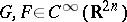. Consideringwith the symplectic structure given by the standard symplectic form (see [a1]),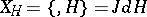is the Hamiltonian vector field generated by.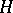is said to be in normal form up to orderwith respect to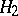if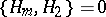for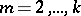.can be transformed into normal form using transformations of the type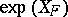. These transformations can be considered as the time-flow of the vector field, and therefore as symplectic diffeomorphisms on. They can also be considered as differential operators acting on the space of homogeneous polynomials of degree. These two points of view are related by the fact that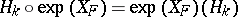. Applying a transformation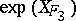with generating function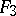homogeneous of degree three givesThe terms of degree three are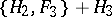. Consequently all terms inthat are in the image of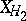can be removed by making the appropriate choice for the generating function. After having made a choice forone getswith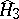in some complement of.

Next, consider a transformation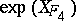now taking a generating function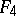homogeneous of degree four. This givesThus, now one can remove all terms in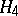that are in the image of. Repeating this process means that up to arbitrary degree one can remove all terms ofthat are in the image of. This leads to the following idea of normal form: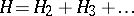is in normal form up to degreewith respect toif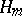,, are in some complement of. When the linearized system is diagonalizable,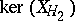can be chosen as a complement to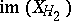, giving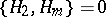,. Letting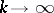, one gets a formal normal form.

More on the basic ideas sketched above can be found in [a10], [a1], [a2]. The above idea was first used, although in an implicit way, by G.D. Birkhoff [a3] for deriving a normal form in the non-resonant case. Attention was again drawn to normal forms by F.G. Gustavson's paper [a7], where he obtained a formal normal form for the resonant cases. A similar normal form was obtained earlier by J. Moser [a9]. Gustavson emphasized that by normalizing up to infinite order extra formal integrals are obtained. The normal form has more symmetry than the original system.

The above ideas have been extended to the case where the linearized system has purely imaginary eigenvalues but is not diagonalizable [a8]. A normal form theory for non–Hamiltonian vector fields has also been developed along the above lines by using the Lie bracket of vector fields rather than Poisson brackets of functions [a4], [a6]. The most general context to formulate the theory is that of reductively filtered Lie algebras [a11].

Normal forms are of importance in the qualitative theory of differential equations. In particular, they play a role in bifurcation theory. Using Lyapunov–Schmidt reduction and the theory of singularities of differentiable mappings one can determine which number of terms of the normal form is sufficient to describe the bifurcation of stationary points and periodic solutions up to topological equivalence [a5], [a6], [a8].

How to Cite This Entry:
Birkhoff normal form. Encyclopedia of Mathematics. URL: http://encyclopediaofmath.org/index.php?title=Birkhoff_normal_form&oldid=17284
This article was adapted from an original article by J.C. van der Meer (originator), which appeared in Encyclopedia of Mathematics - ISBN 1402006098. See original article